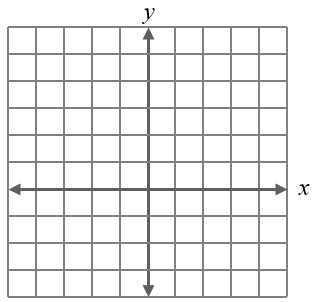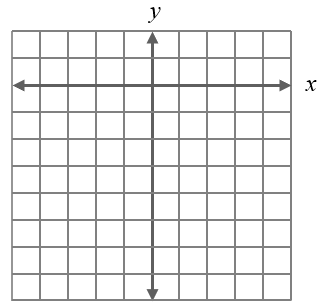# Practice Graphing Lines

### Part 1:

Graph the following equations.

 1. $$y=3x-8$$2. $$y=-\dfrac{4}{3}x+5$$3. $$y=x-7$$4. $$y=-4$$5. $$y=4+\dfrac{2}{3}x$$6. $$x=-3$$### Part 2:

Write an equation that best represents the points contained in each line below in slope-intercept form.

 7.8.9.